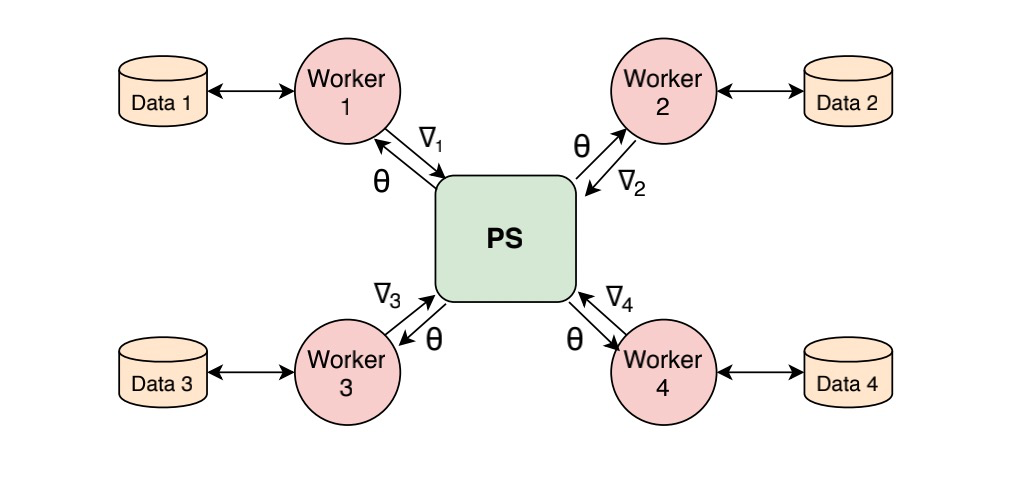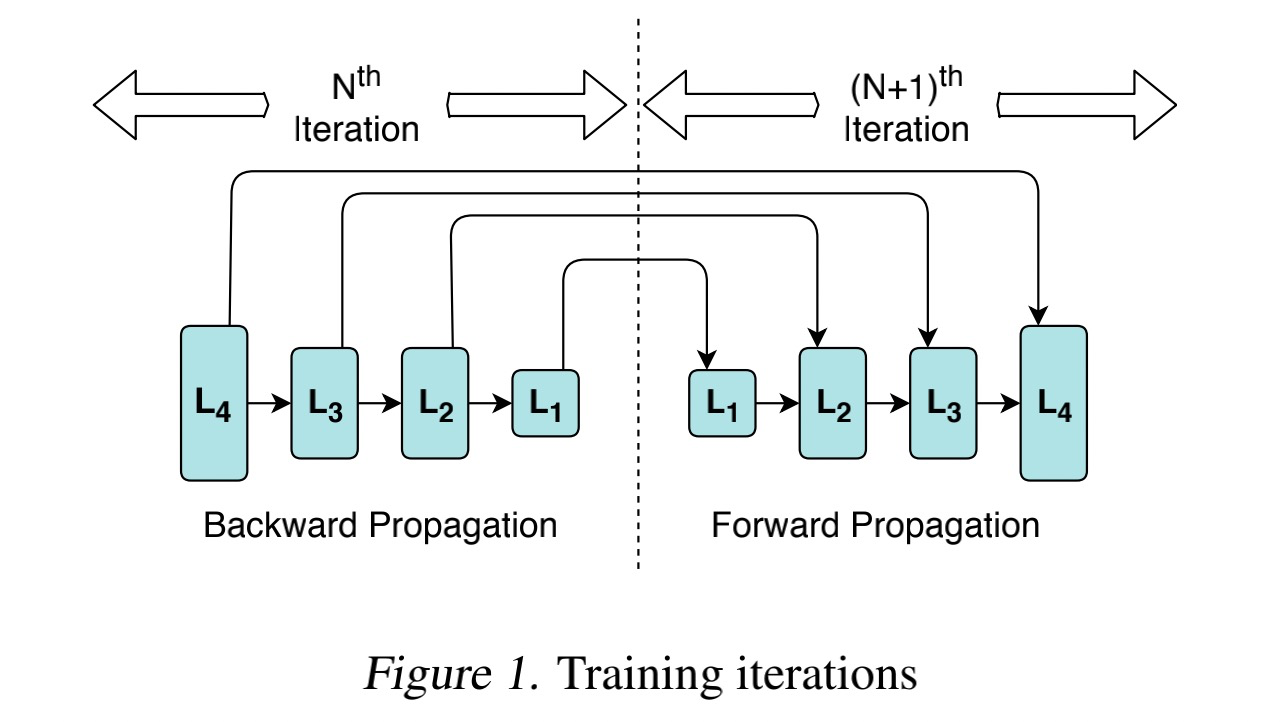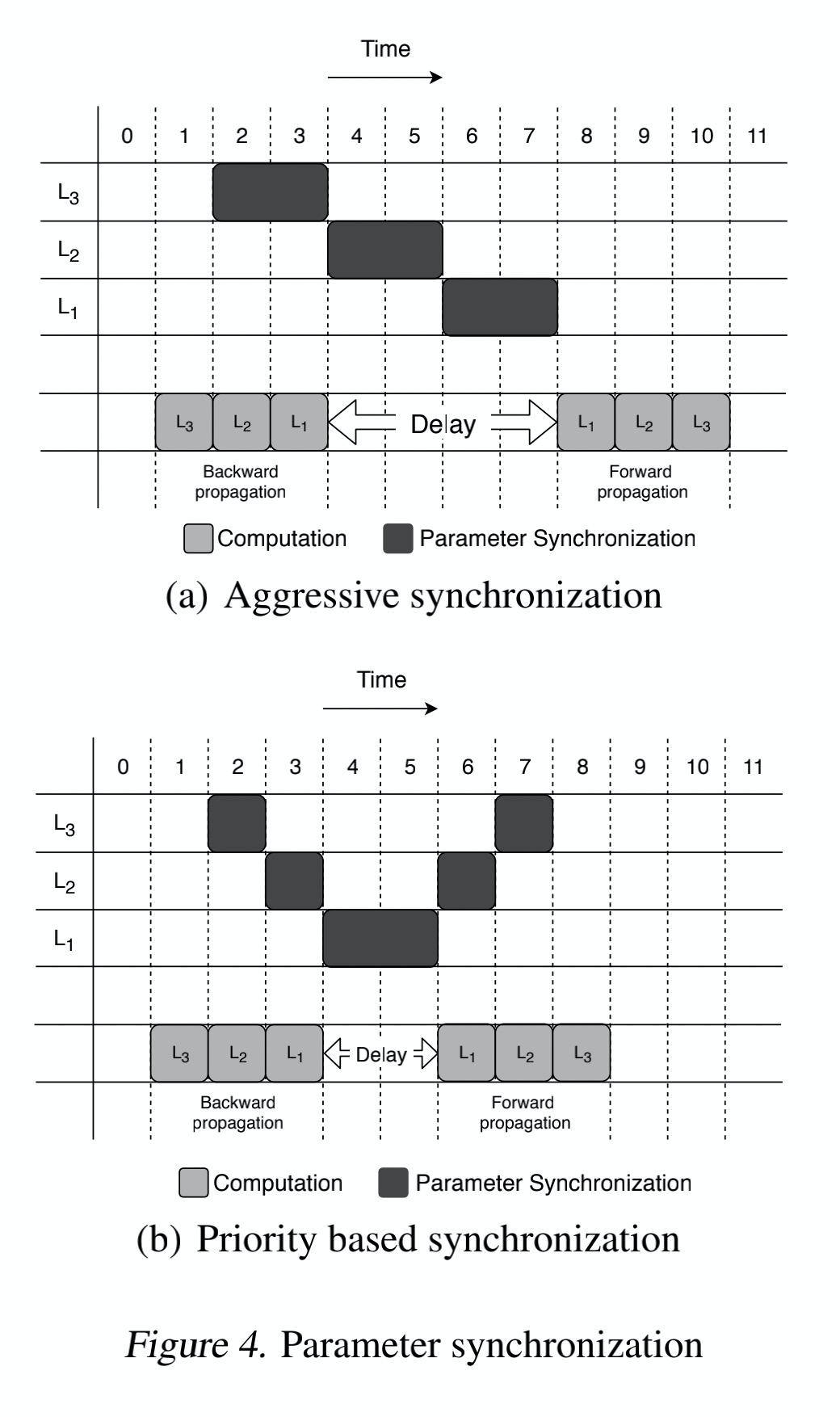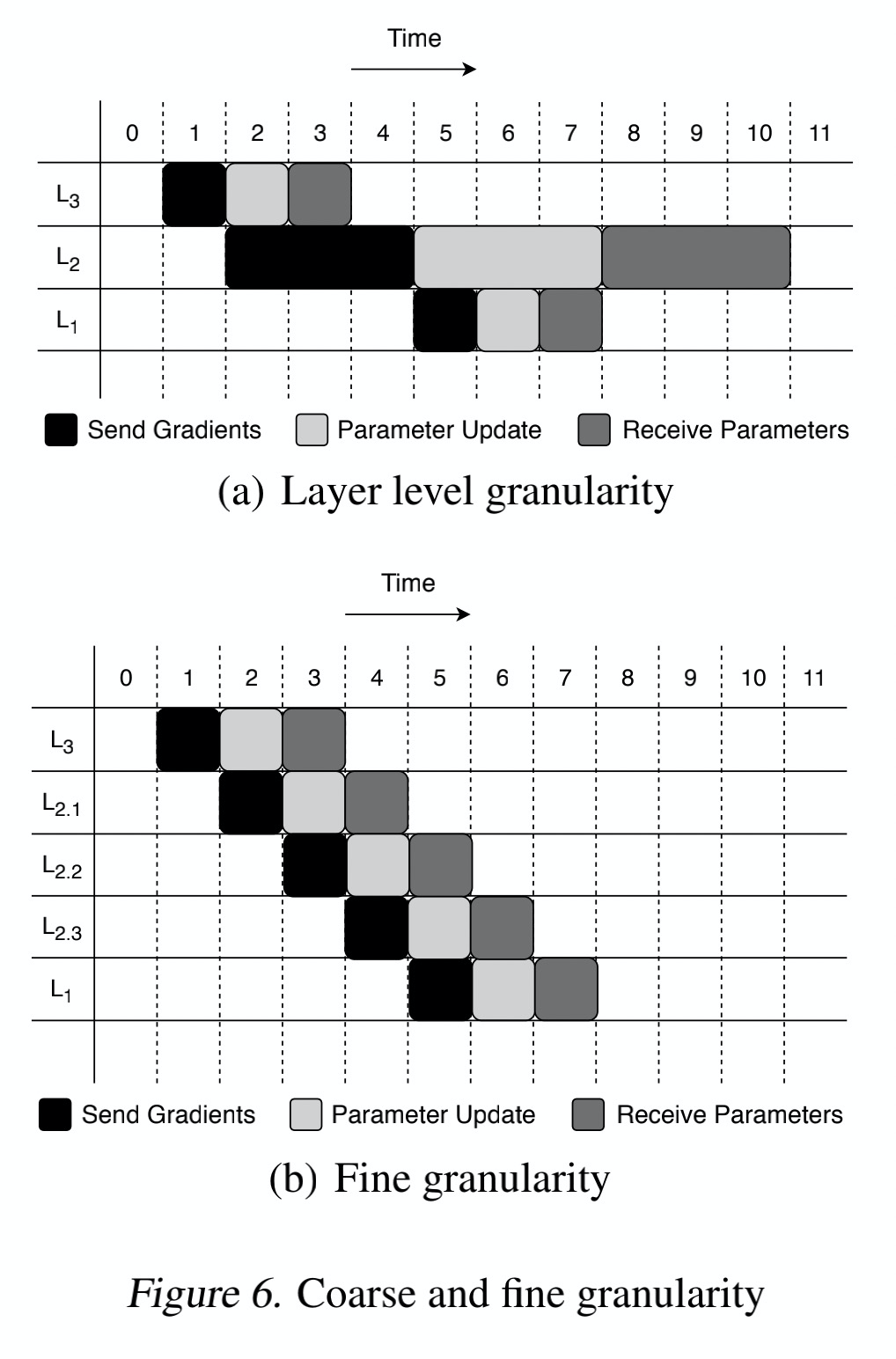# Parameter priority propagation method in neural network distributed training

Time：2021-1-13

## Parameter priority propagation method in distributed training of technical blog neural network

Author: Ni Hao

This paper is from the parallel & distributed learning section of the 2019 SysML conference.

Data parallel training has been widely used in distributed computing of deep neural networks. However, the performance improvement of distributed computing is often limited by the bottleneck of parameter synchronization. The authors and others proposed a new parameter synchronization mechanism: priority based parameter propagation (P3), which improves the utilization of network bandwidth by the training cluster of the model and speeds up the training speed of the model.
Let’s first review the distributed training of neural networks.

### Distributed training of deep neural network

There are usually two strategies for distributed training of neural networks. One is model parallelism, which means that different machines in the distributed system are responsible for computing in different parts of a single network, that is, putting different layers of the model on different work nodes. When the model is too large, its computing efficiency is not high. The other is data parallel. Different machines have a complete copy of the whole model. Each machine only obtains different parts of the whole data. That is to say, the training data is divided into many blocks and distributed to different worker nodes. Each node calculates separately, but shares a model. The calculation results are combined by some methods. Of course, the two strategies are not in conflict and can be mixed.

The common architecture of data parallel is parameter server, which is PS architecture.

There are two parallel modes of data parallel: synchronous training and asynchronous training. Synchronous training means that the gradient of all worker nodes is calculated and updated uniformly. Asynchronous training means that the worker node computes the model parameters independently from the PS node, calculates the gradient independently and updates it. Data parallel training using synchronous random gradient descent (SGD) algorithm is a very popular method.The work of each node can be divided into three steps

1. Nodes obtain the latest model parameters from PS nodes and propagate the training data they are responsible for.
2. Each node independently back propagates the training data of different parts to get the gradient of each model parameter.
3. The gradient calculation results of each worker node are synchronized, and the parameters of the model are updated

And then it iterates over and over again.

Every iteration of a node needs to synchronize a large number of parameters through the network, which puts forward a very high requirement for network bandwidth. One way to solve this problem is to increase the bandwidth of the network, but it means a very high cost. At the same time, with the increase of the size of the model, the cost is immeasurable. Therefore, we should seek the solution under the limited bandwidth.

In recent years, a popular scheme is gradient compression, but the disadvantage of this scheme is that some information is lost, which may affect the accuracy of the model.

Another way is to improve the utilization of network bandwidth. In the process of training, if we synchronize the gradient after each iteration, the network traffic will increase explosively, while in the process of worker node calculation, the network is basically idle. Therefore, we can send the gradient that has been calculated to the PS node when the worker node calculates the gradient of other layers, that is, the communication between the worker node and the PS node and the back propagation of the worker node can be carried out at the same time, so that the network bandwidth can be used more effectively. Some deep learning frameworks have achieved this, such as tensorflow, mxnet and caffe2.

### Limitation and optimization

In their work, the authors found that there are still some limitations in the above schemes. Through optimization, the utilization rate of network bandwidth and the calculation speed of the model can be further improved.

#### Priority of parameter transfer in back propagation

In the training of neural network model, we usually do the following: back propagation – forward propagation with the parameters obtained from back propagation. To be more detailed, back propagation calculation is started from the last layer, and then forward propagation is started from the first layer, as shown in the figure below.In the nth iteration, we start from the output layer, calculate the gradient according to loss and update the parameters of L4 layer, then recursively calculate the gradient of the input layer, that is, L1 layer, and update the parameters of the whole model at the same time. Then we start from the input layer and perform n + 1 In the next iteration, the parameters of the updated model are calculated layer by layer until the results of the output layer are obtained, and then back propagation is performed.

We can observe that in the process of iteration, the first calculated parameter (L4 layer parameter) is always used last, while the last calculated parameter of back propagation is used first by forward propagation. We find that in the process of neural network calculation, the interval between the parameters of each layer from obtaining to using is different, and the larger the interval is when the number of layers is close to the output layer. In the past distributed training, parameters often start to synchronize at the end of a layer’s back propagation, which may lead to L2 layer’s parameters not being synchronized, but L1 layer has finished back propagation, so we have to wait for L2 layer to complete synchronization, L1 layer to complete parameter synchronization, and then accept PS node’s parameter update to start L1 layer’s forward propagation. In this way, the gap between forward propagation and backward propagation will be too large.

Therefore, we think that in parameter synchronization, the layer with lower layer number should have higher priority than the layer with higher layer number.(a) in the figure above represents the previous synchronization mechanism. We improve it by preferentially synchronizing the gradient of the lower layer. As shown in (b), when the gradient calculation of the L1 layer is completed, but the synchronization of the parameters of the higher layer has not been completed, the L1 layer is preferentially synchronized In this way, the interval between back propagation and forward propagation is shortened, but the network load is not increased.

#### Granularity selection in parameter synchronization

The communication time for parameter synchronization is mainly composed of three parts

1. Time of gradient transmission from worker node to PS node
2. Time required for PS node to update model parameters using gradient
3. PS node sends the updated parameters to each worker node

As described before, in the past, we used to synchronize parameters with layer granularity. As shown in figure (a), we can make full use of the idle network bandwidth and shorten the communication time by implementing data transmission and parallel execution of computation.

However, in the model, if there are many parameters in one layer, such as L2 in figure (a), it takes three times as long as L1 and L3 to complete the parameter synchronization in each step, we will find that it will slow down the parameter synchronization, or “block” the parameter transmission. For example, when the time is 4, there is only gradient transmission, but no parameter update calculation. This is because our parameter synchronization is based on layers. The update of parameters in each layer must wait until the gradients of all nodes in the layer are transmitted, but we do not need to wait until the gradients of all nodes are received before updating parameters.Therefore, we can adopt a smaller granularity of parameter synchronization. For example, divide the L2 layer parameters into three parts and synchronize them separately, as shown in (b). By adopting smaller granularity, we can improve bandwidth utilization as much as possible, which is to increase the coincidence degree between different layers in the graph.

### summary

The above two points are the optimization method adopted by P3. The author implements this mechanism on the basis of mxnet. Students who are interested can visit GitHub to read the relevant source code. According to the author’s test, the training efficiency of P3, resnet-50, sockeye, vgg-19 and other models increased by 25%, 38% and 66% respectively. The advantage of this synchronization mechanism is that it does not conflict with other optimization methods, and does not affect the accuracy of the model. However, the improvement of the training efficiency of the distributed model is limited, and not all models have obvious improvement, especially when the model is small or the network bandwidth is very limited.

### reference

• https://mlsys.org/Conferences/2019/doc/2019/75.pdf
• https://github.com/anandj91/p3

## General method of Tkinter (21) components

method explain after(delay_ms, callback=None, *args) At least delay_ Ms after calling callback, no callback, equivalent time.sleep (); returns an ID to cancel after_ The cancel () method uses after_cancel(id) Cancel the callback of after method call after_idle(func, *args) Similar to the after method, but called when there is no event idle bell() A beep bind(sequence=None, […]